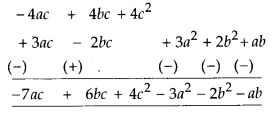# MP Board Class 8th Maths Solutions Chapter 9 Algebraic Expressions and Identities Ex 9.3

## MP Board Class 8th Maths Solutions Chapter 9 Algebraic Expressions and Identities Ex 9.3

Question 1.
Carry out the multiplication of the expressions in each of the following pairs.
(i) 4p, q + r
(ii) ab, a – b
(iii) a + b, 7a2b2
(iv) a2 – 9, 4a
(v) pq + qr + rp, 0
Solution:
(i) 4p × (q + r) = 4p × q + 4p × y = 4pq + 4pr
(ii) ab × (a – b) = a2b – ab2
(iii) (a + b) × 7a2b2 = 7a3b2 + 7a2b3
(iv) (a2 – 9) × (4a) = 4a3 – 36a
(v) (pq + qr + rp) × 0 = 0

Question 2.
Complete the table.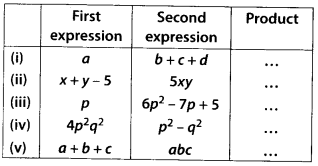Solution: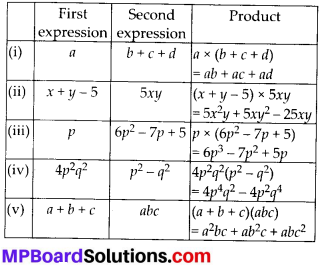Question 3.
Find the product.
(i) (a2) × (2a22) × (4a26)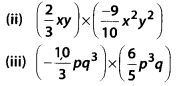(iv) x × x2 × x3 × x4
Solution: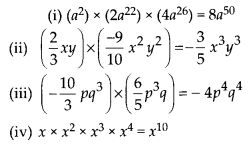Question 4.
(a) Simplify 3x(4x – 5) + 3 and find its values for
(i) x = 3
(ii) x = $$\frac{1}{2}$$
(b) Simplify a(a2 + a + 1) + 5 and find its value for
(i) o = 0
(ii) a = 1
(iii) a = – 1
Solution:
(a) 3x(4x – 5) + 3 = 12x2 – 15x + 3
(i) For x = 3,
12(3)2 – 15(3) + 3 = 108 – 45 + 3 = 66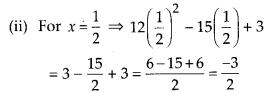(b) a(a2 + a + 1) + 5 = a3 + a2 + a + 5
(i) For a = 0, (0)3 + 02 + 0 + 5 = 5
(ii) For a = 1, 13 + 12 + 1 + 5 = 8
(iii) For a = -1, (-1)3 + (-1)2 + (-1) + 5 = -1 + 1 – 1 + 5 = 4Question 5.
(a) Add: p(p – q), q(q – r) and r(r – p)
(b) Add : 2x(z – x – y) and 2y(z – y – x)
(c) Subtract: 3l(l -4m + 5n) from 4l(10n – 3m + 2l)
(d) Subtract: 3o(a + b + c) – 2b(a – b + c) from 4c(-a + b + c)
Solution:
(a) First expression
= p(p – q) = p2 – pq
Second expression = q(q- r) = q2 – qr… (ii)
Third expression = r(r – p) = r2 – pr ….(iii)
Adding (i), (ii) and (iii), we get
p2 – pq + q2 – qr + r2 – pr = p2 + q2 + r2 – pq – qr – pr

(b) First expression = 2x(z -x-y)
= 2xz – 2x2 – 2xy
Second expression = 2y(z -y-x)
= 2yz – 2y2 – 2xy
Adding the two expressions, we get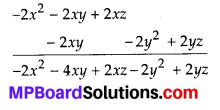(c) First expression = 3l(l – 4m + 5n)
= 3l2 – 121m + 15In
Second expression = 4l(10n – 3m + 2l)
= 40ln – 12lm + 8l2
Subtracting the two expressions, we get 40/n –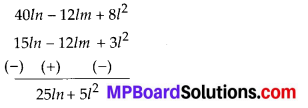(d)
First expression= 3a(a + b + c) – 2b(a – b + c)
= 3a2+3ab + 3ac – 2ab + 2b2 – 2bc
= 3a2 + ab + 2b2 + 3ac – 2bc
Second expression = 4c(- a + b + c)
= – 4ac + 4bc + 4c2
Subtracting the two expressions, we get